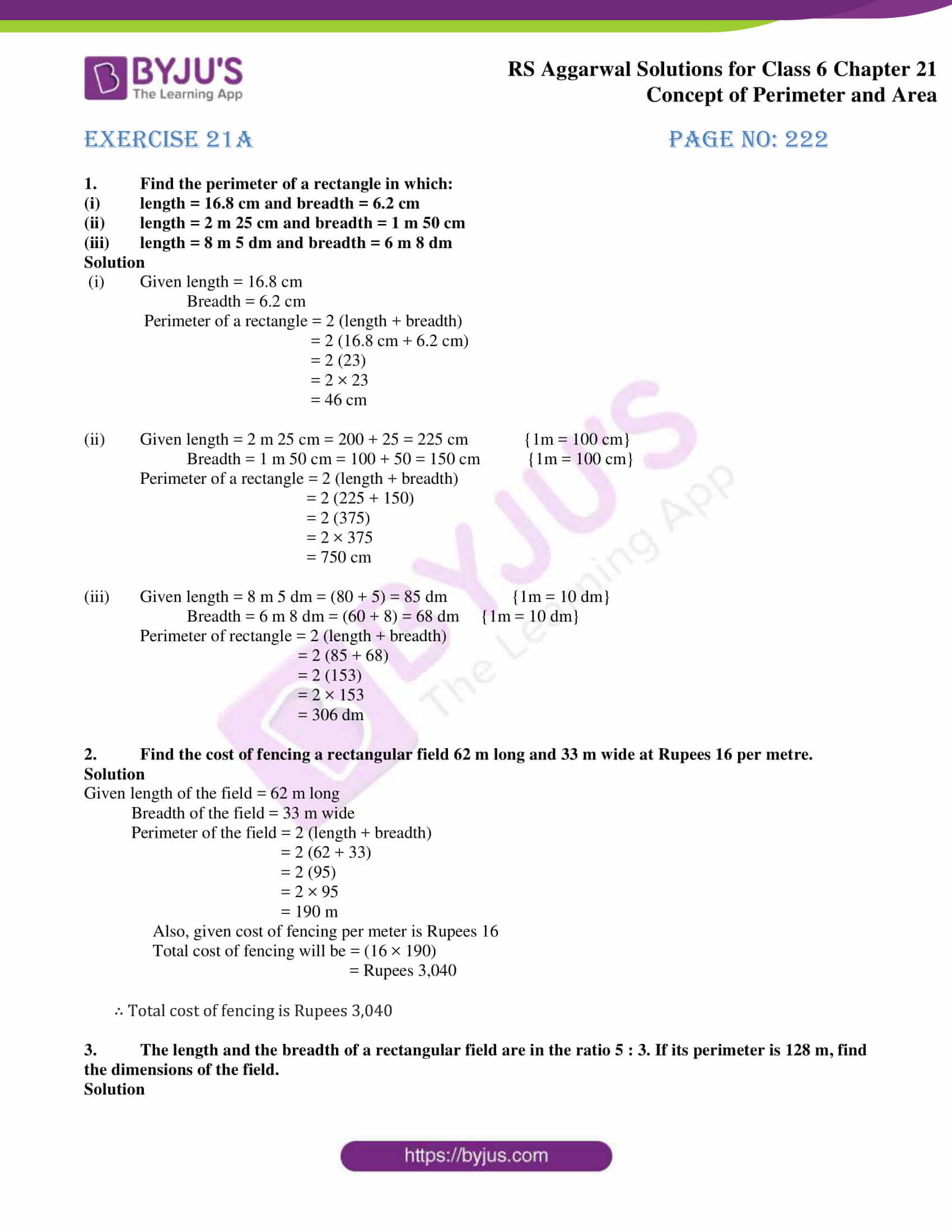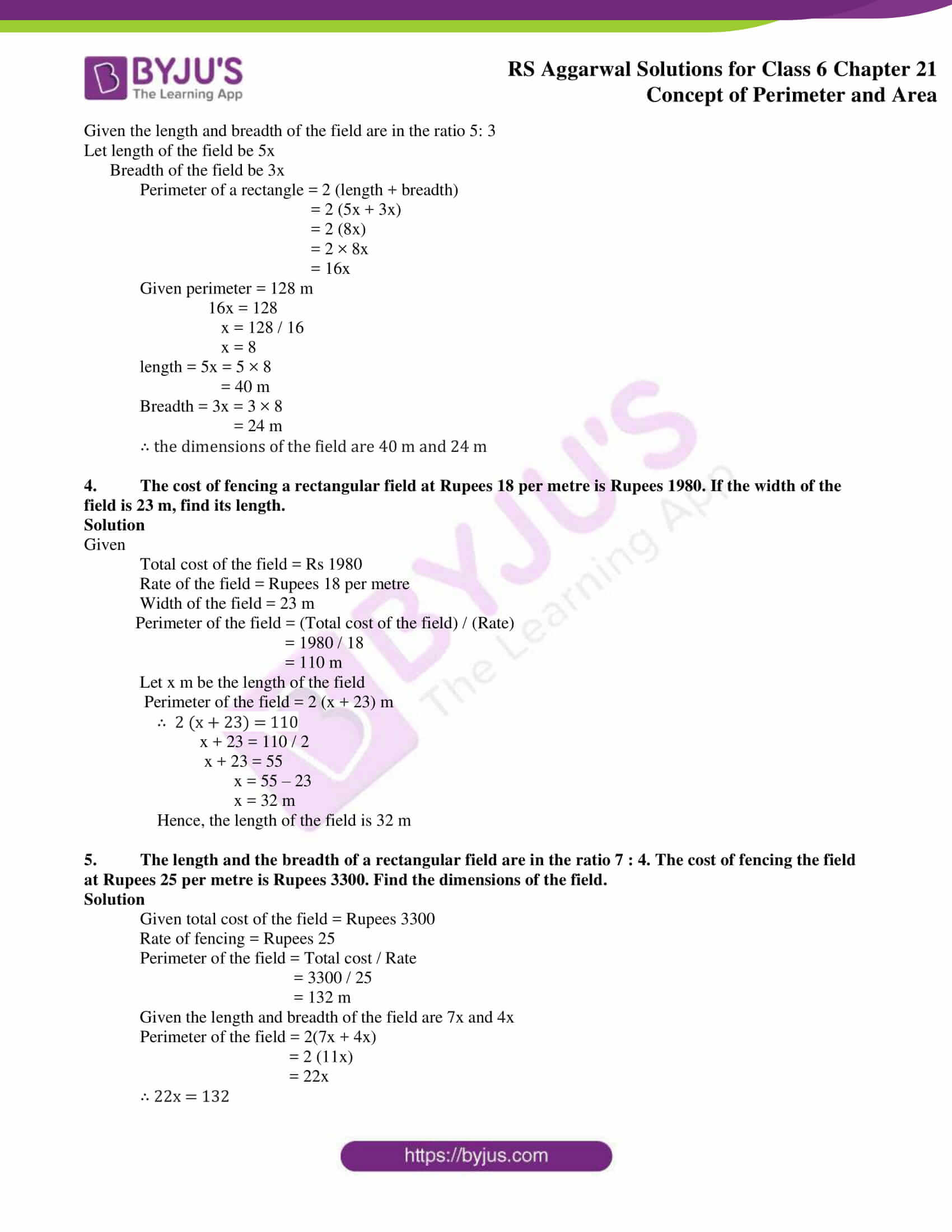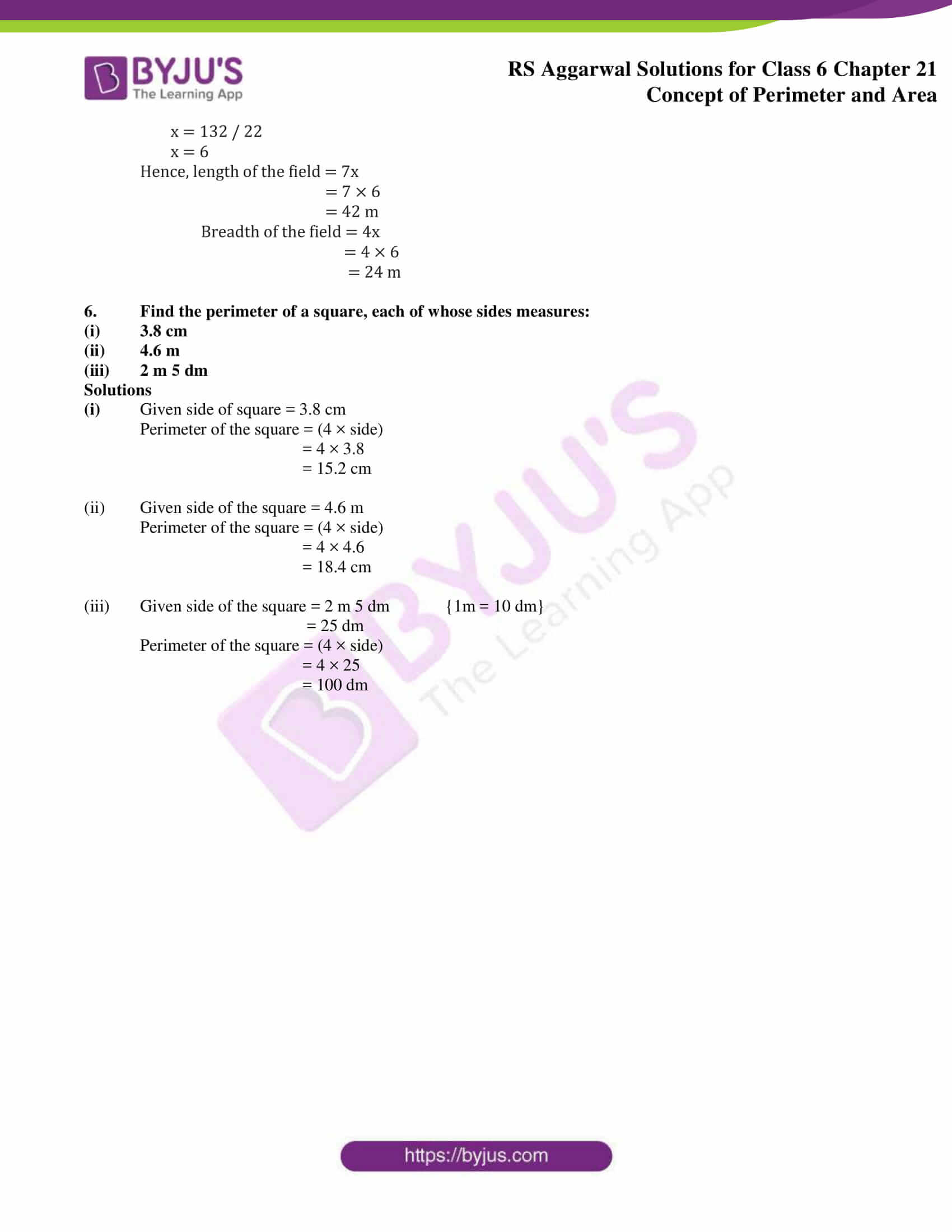# RS Aggarwal Solutions for Class 6 Chapter 21 Concept of Perimeter and Area Exercise 21A

RS Aggarwal Solutions are designed by highly experienced faculty to help students excel in their exams. The necessary formulas and concepts which are important are highlighted to provide a better learning experience. This exercise mainly has various formulas which are used in finding the perimeter of a plane figure, rectangle and a square along with the steps used in solving them. RS Aggarwal Solutions for Class 6 Chapter 21 Concept of Perimeter and Area Exercise 21A PDF are provided here.

## Download PDF of RS Aggarwal Solutions for Class 6 Chapter 21 Concept of Perimeter and Area Exercise 21A### Access answers to Maths RS Aggarwal Solutions for Class 6 Chapter 21 Concept of Perimeter and Area Exercise 21A

1. Find the perimeter of a rectangle in which:

(i) length = 16.8 cm and breadth = 6.2 cm

(ii) length = 2 m 25 cm and breadth = 1 m 50 cm

(iii) length = 8 m 5 dm and breadth = 6 m 8 dm

Solution

(i) Given length = 16.8 cm

Perimeter of a rectangle = 2 (length + breadth)

= 2 (16.8 cm + 6.2 cm)

= 2 (23)

= 2 × 23

= 46 cm

(ii) Given length = 2 m 25 cm = 200 + 25 = 225 cm {1m = 100 cm}

Breadth = 1 m 50 cm = 100 + 50 = 150 cm {1m = 100 cm}

Perimeter of a rectangle = 2 (length + breadth)

= 2 (225 + 150)

= 2 (375)

= 2 × 375

= 750 cm

(iii) Given length = 8 m 5 dm = (80 + 5) = 85 dm {1m = 10 dm}

Breadth = 6 m 8 dm = (60 + 8) = 68 dm {1m = 10 dm}

Perimeter of rectangle = 2 (length + breadth)

= 2 (85 + 68)

= 2 (153)

= 2 × 153

= 306 dm

2. Find the cost of fencing a rectangular field 62 m long and 33 m wide at Rupees 16 per metre.

Solution

Given length of the field = 62 m long

Breadth of the field = 33 m wide

Perimeter of the field = 2 (length + breadth)

= 2 (62 + 33)

= 2 (95)

= 2 × 95

= 190 m

Also, given cost of fencing per meter is Rupees 16

Total cost of fencing will be = (16 × 190)

= Rupees 3,040

∴ Total cost of fencing is Rupees 3,040

3. The length and the breadth of a rectangular field are in the ratio 5 : 3. If its perimeter is 128 m, find the dimensions of the field.

Solution

Given the length and breadth of the field are in the ratio 5: 3

Let length of the field be 5x

Breadth of the field be 3x

Perimeter of a rectangle = 2 (length + breadth)

= 2 (5x + 3x)

= 2 (8x)

= 2 × 8x

= 16x

Given perimeter = 128 m

16x = 128

x = 128 / 16

x = 8

length = 5x = 5 × 8

= 40 m

Breadth = 3x = 3 × 8

= 24 m

∴ the dimensions of the field are 40 m and 24 m

4. The cost of fencing a rectangular field at Rupees 18 per metre is Rupees 1980. If the width of the field is 23 m, find its length.

Solution

Given

Total cost of the field = Rs 1980

Rate of the field = Rupees 18 per metre

Width of the field = 23 m

Perimeter of the field = (Total cost of the field) / (Rate)

= 1980 / 18

= 110 m

Let x m be the length of the field

Perimeter of the field = 2 (x + 23) m

∴ 2 (x + 23) = 110

x + 23 = 110 / 2

x + 23 = 55

x = 55 – 23

x = 32 m

Hence, the length of the field is 32 m

5. The length and the breadth of a rectangular field are in the ratio 7 : 4. The cost of fencing the field at Rupees 25 per metre is Rupees 3300. Find the dimensions of the field.

Solution

Given total cost of the field = Rupees 3300

Rate of fencing = Rupees 25

Perimeter of the field = Total cost / Rate

= 3300 / 25

= 132 m

Given the length and breadth of the field are 7x and 4x

Perimeter of the field = 2(7x + 4x)

= 2 (11x)

= 22x

∴ 22x = 132

x = 132 / 22

x = 6

Hence, length of the field = 7x

= 7 × 6

= 42 m

Breadth of the field = 4x

= 4 × 6

= 24 m

6. Find the perimeter of a square, each of whose sides measures:

(i) 3.8 cm

(ii) 4.6 m

(iii) 2 m 5 dm

Solutions

(i) Given side of square = 3.8 cm

Perimeter of the square = (4 × side)

= 4 × 3.8

= 15.2 cm

(ii) Given side of the square = 4.6 m

Perimeter of the square = (4 × side)

= 4 × 4.6

= 18.4 cm

(iii) Given side of the square = 2 m 5 dm {1m = 10 dm}

= 25 dm

Perimeter of the square = (4 × side)

= 4 × 25

= 100 dm

### Access other exercises of RS Aggarwal Solutions for Class 6 Chapter 21 Concept of Perimeter and Area

Exercise 21E Solutions